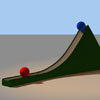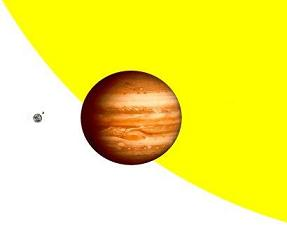#### You may also like### Lunar Leaper

Gravity on the Moon is about 1/6th that on the Earth. A pole-vaulter 2 metres tall can clear a 5 metres pole on the Earth. How high a pole could he clear on the Moon?### Which Twin Is Older?

A simplified account of special relativity and the twins paradox.### Whoosh

A ball whooshes down a slide and hits another ball which flies off the slide horizontally as a projectile. How far does it go?

# Escape from Planet Earth

##### Age 16 to 18Challenge Level

A cannon ball is shot vertically upwards with speed v. How fast would the ball need to go such that it would never fall back to Earth?

How fast would you need to fire the cannon ball to escape from the moon, Jupiter or the surface of the sun? Before you try these parts of the calculation, do you think that these escape velocities will be lower or higher than that of Earth in each case? To get a feel for the relative sizes, you can look at this scale picture*.Suppose that I go to planet Tiny, which is the same mass as Earth but a lot smaller. I fire a cannon ball again at 50% of the speed of light. How small would planet Tiny need to be so that the cannon ball cannot escape to infinity?

Supposing now that I go to planet Heavy, which is the same size as Earth but a lot more dense, and fire my cannon ball up at 50% of the speed of light. How many times more dense than Earth would planet Heavy need to be so that the cannon ball cannot escape its gravity?

Compare these results to trying to escape from a typical Neutron star which would weigh around twice the mass of the sun and have a radius of only 10km (this is around the same density as trying to squeeze the entire earth into a ball of 100m radius).

NOTES AND BACKGROUND

This problem introduces the concept of Escape Velocity . Whilst escape velocity is a real concept, attempting to reach such speeds in the atmosphere would cause immediate disintegration of almost any object. It was noted in the 1700s by Laplace that for a very massive body the escape velocity would approach the speed of light. This concept was developed in the 1920s following Einstein's discovery of general relativity. The black hole was discovered as a concept: an object so dense that even light beams would not be able to escape its gravitational pull. Over the last couple of decades evidence for the real existence of black holes in the galaxy has grown.

 Name Diameter relative to earth Mass relative to earth Jupiter 11.209 317.8 Moon 0.273 0.0123 The sun 109 332946

In this problem you will need to know that the gravitational potential energy of an object of mass m at a distance r from the centre of an object of mass M is given by PE = GMm/r where $G = 6.674\times 10^{-11}m^3kg^{-1}s^{-2}$ is Newton's gravitational constant. $M= 5.9763\times 10^{24}kg$ is the mass of the earth and the radius of the earth is 6378km

* Image adapted from http://commons.wikimedia.org/wiki/Image:Portrait_de_famille_%281_px_%3D_1000_km%29.jpg